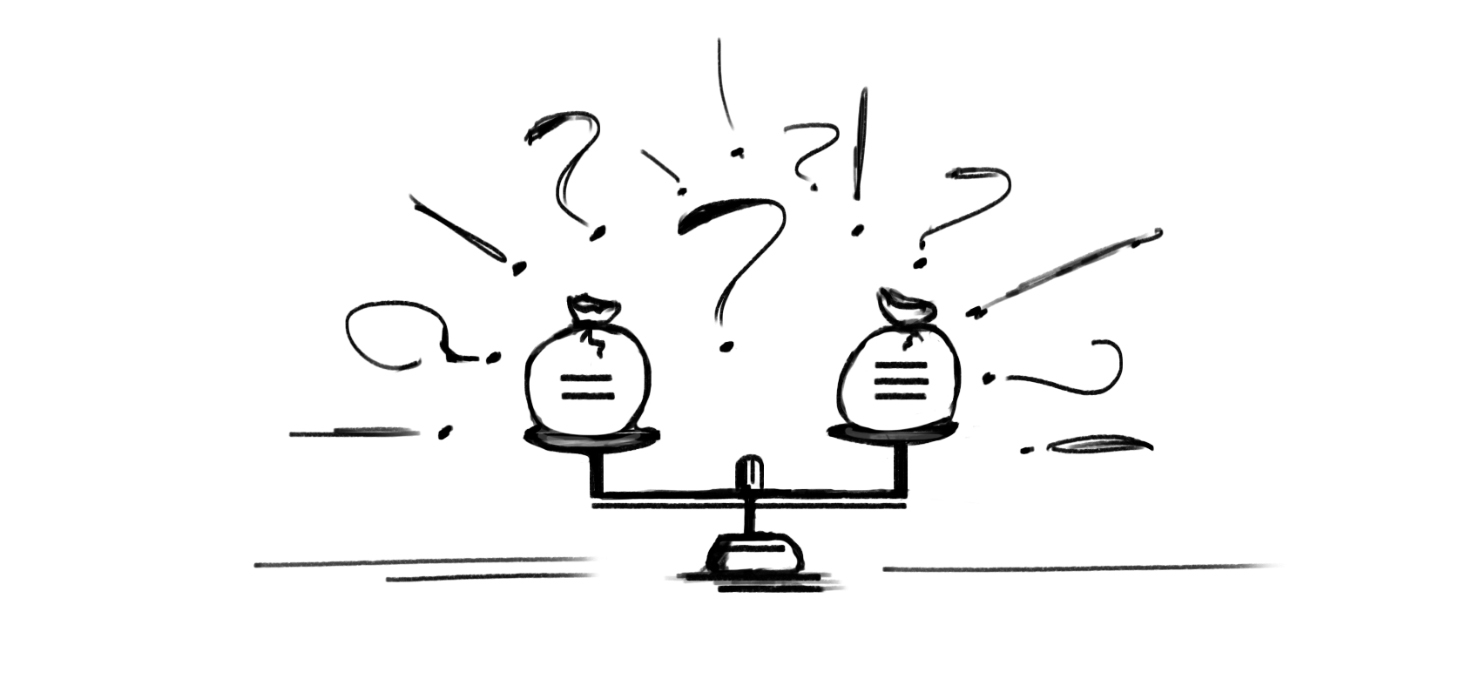# Strict equality~ Basics ~I’m writing JavaScript for a long time, and one of the first things that I learned is to use === (triple equals) instead of == (double equals). It is the be-on-the-safe-side mental model. For example:

``````user.id === id
``````

over

``````user.id == id
``````

Such equality comparisons are a source of a lot of jokes. Very often, we stumble on statements that look illogical. For example, what if we compare an empty string with a boolean `false`:

``````console.log("" == false); // true
``````

This comparison is `true` because we use double equals or the so-called loose equality. The result is `true` because both values are converted to a common type and then compared. The above, in reality, looks more like:

``````console.log(Boolean("") === false); // true
``````

After the conversion JavaScript engine uses `===` (strict equality).

So, use triple equals to make your code explicit. It will save you from some weird bugs.